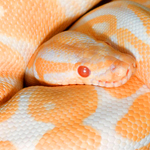# Python 2.7 Tutorial Pt 3In part 3 of my Python 2.7 Tutorial, I explain how to use the Dictionary, Print method and how to work with String methods.

If you haven’t seen part 1 or 2 definitely look at them first or you’ll be very confused Python 2.7 Tutorial Part 1 and Python 2.7 Tutorial Part 2.

All of the code I use in these tutorials follow the video and can be used however you’d like.

If you have any questions or comments leave them below.

Here is the Code for this Python Tutorial

#! /usr/bin/python

import math

# Formatting output with the print function
strName = ‘Bob’
floatAge = 35.4
charSex = ‘M’
intKids = 2
boolMarried = True

print ‘%s is %f years old’ % (strName, floatAge)
print ‘Sex: %c’ % (charSex)
print ‘He has %d kids and said it\’s %s he is married’ % (intKids,boolMarried)

print ‘%s is %.1f years old’ % (strName, floatAge)

# Formatting Output Further
print ‘%.15f’ %(math.pi)
print ‘%20f’ %(math.pi)
print ‘%20.15f’ %(math.pi)
print ‘%-25.15f is the value of pi’ %(math.pi)

precisionPi = int(raw_input(“How precise should pi be: “))
print ‘PI = %.*f’ % (precisionPi, math.pi)

# A Bunch of String Methods

bigString = ‘Here is a long string that I will be messing with’

print bigString[1:20:2]

print bigString.find(‘string’)
print bigString.find(‘massive’)

print bigString.count(‘e’)
print bigString.count(‘e’, 4)
print bigString.count(‘e’, 0, 20)

copyStr = tuple(bigString)
print copyStr
print ”.join(copyStr)
print ‘, ‘.join(copyStr)

print bigString.lower()
print bigString.upper()

print bigString.replace(‘long’,’small’)

print bigString.split(‘ ‘)

randomWhite = ‘       This string has random white space      ‘
print randomWhite.strip()